## Tamilnadu Samacheer Kalvi 11th Maths Solutions Chapter 7 Matrices and Determinants Ex 7.5

Choose the correct or the most suitable answer from the given four alternatives.

Question 1.
If aij = $$\frac{1}{2}$$ (3i – 2j) and A = [aij]2×2 is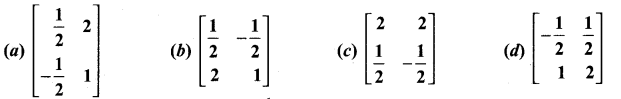Solution: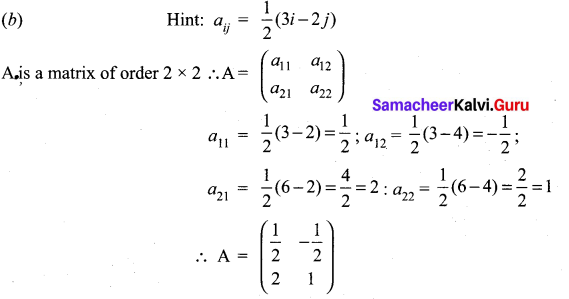Question 2.
What must be the matrix X, if 2X + $$\left[\begin{array}{ll}{1} & {2} \\ {3} & {4}\end{array}\right]=\left[\begin{array}{ll}{3} & {8} \\ {7} & {2}\end{array}\right]$$ ?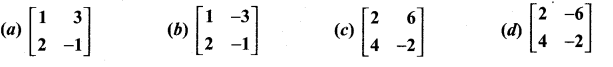Solution: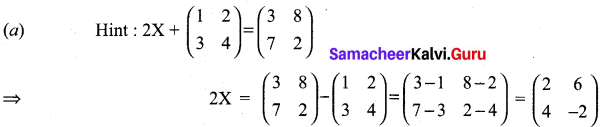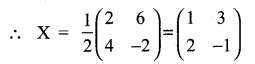Question 3.
Which one of the following is not true about the matrix $$\left[\begin{array}{lll}{1} & {0} & {0} \\ {0} & {0} & {0} \\ {0} & {0} & {5}\end{array}\right]$$?
(a) a scalar matrix
(b) a diagonal matrix
(c) an upper triangular matrix
(d) A lower triangular matrix
Solution:
(b) a diagonal matrixQuestion 4.
If A and B are two matrices such that A + B and AB are both defined, then …………
(a) A and B are two matrices not necessarily of same order.
(b) A and B are square matrices of same order.
(c) Number of columns of a is equal to the number of rows of B.
(d) A = B.
Solution:
(b) A and B are square matrices of same order.

Question 5.
If A = $$\left[\begin{array}{rr}{\lambda} & {1} \\ {-1} & {-\lambda}\end{array}\right]$$, then for what value of λ, A2 = 0?
(a) 0
(b) ±1
(c) -1
(d) 1
Solution: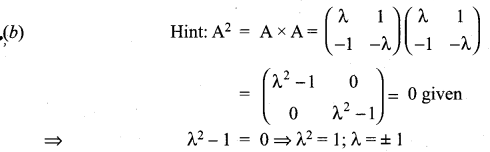Question 6.
If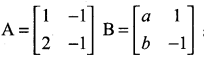and (A + B)2 = A2 + B2, then the values of a and b are ……………….
(a) a = 4, b = 1
(b) a = 1, b = 4
(c) a = 0, b = 4
(d) a = 2, b = 4
Solution: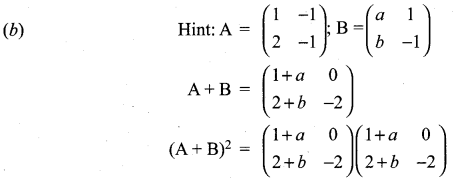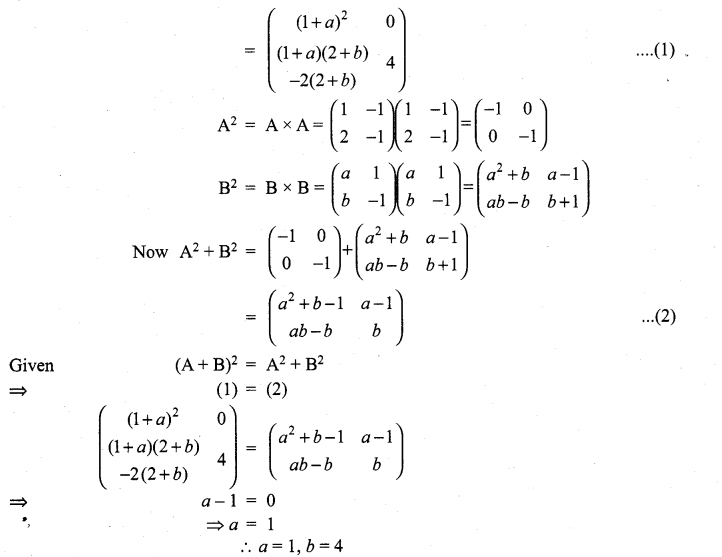Question 7.
If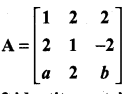is a matrix satisfying the equation AAT = 9I, where I is 3 × 3 identity matrix, then the ordered pair (a, b) is equal to ………….
(a) (2, -1)
(b) (-2, 1)
(c) (2, 1)
(d) (-2, -1)
Solution: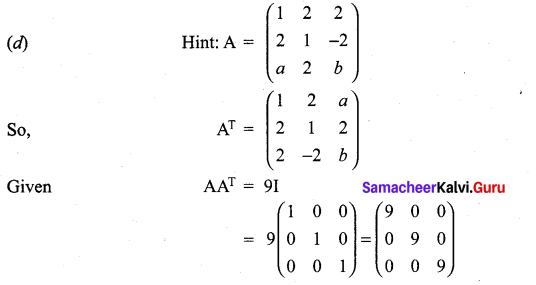Question 8.
If A is a square matrix, then which of the following is not symmetric?
(a) A + AT
(b) AAT
(c) ATA
(d)A – AT
Solution:
(b)

Question 9.
If A and B are symmetric matrices of order n, where (A ≠ B), then …………….
(a) A + B is skew-symmetric
(b) A + B is symmetric
(c) A + B is a diagonal matrix
(d) A + B is a zero matrix
Solution:
(b)Question 10.
If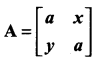and if xy = 1, then det (AAT) is equal to …………..
(a) (a – 1)2
(b) (a2 + 1)2
(c) a2 – 1
(d) (a2 – 1)2
Solution: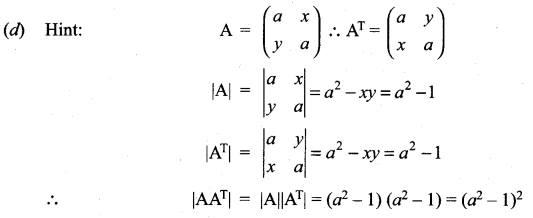Question 11.
The value of x, for which the matrix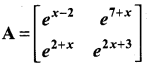is singular is ………….
(a) 9
(b) 8
(c) 7
(d) 6
Solution:
(b) Hint: Given A is a singular matrix ⇒ |A| = 0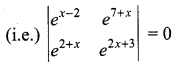⇒ ex-2.e2x+3 – e2+x.e7+x = 0
⇒ e3x+1 – e9+2x = 0 ⇒ e3x+1 = e9+2x
⇒ 3x + 1 = 9 + 2x
3x – 2x = 9 – 1 ⇒ x = 8

Question 12.
If the points (x, -2), (5, 2), (8, 8) are collinear, then x is equal to …………
(a) -3
(b) $$\frac{1}{3}$$
(c) 1
(d) 3
Solution:
(d) Hint: Given that the points are collinear
So, area of the triangle formed by the points = 0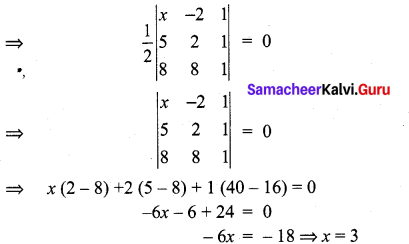Question 13.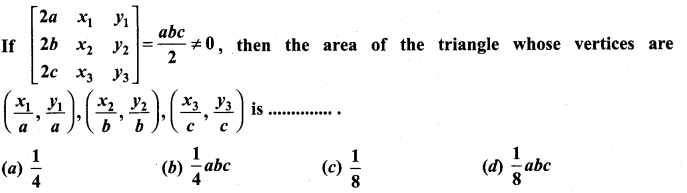Solution: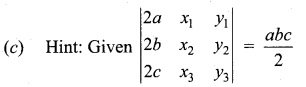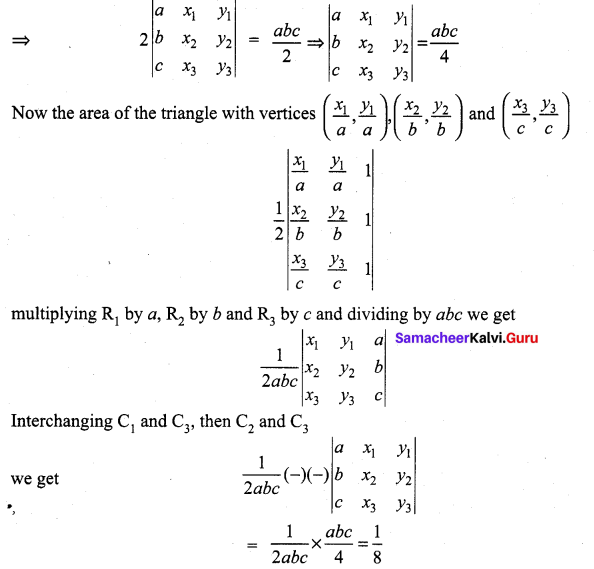Question 14.
If the square of the matrix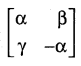is the unit matrix of order 2, then α, β and γ should satisfy the relation.
(a) 1 + α2 + βγ = 0
(b) 1 – α2 – βγ = 0
(c) 1 – α2 + βγ = 0
(d) 1 + α2 – βγ = 0
Solution: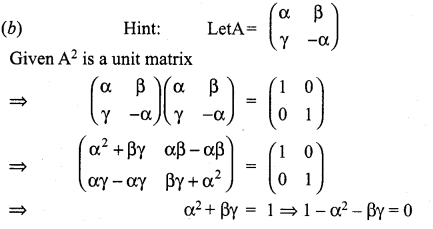Question 15.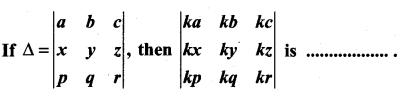(a) Δ
(b) kΔ
(c) 3kΔ
(d) k3Δ
Solution: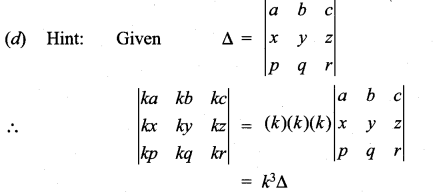Question 16.
A root of the equation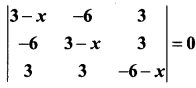is …………….
(a) 6
(b) 3
(c) 0
(d) -6
Solution: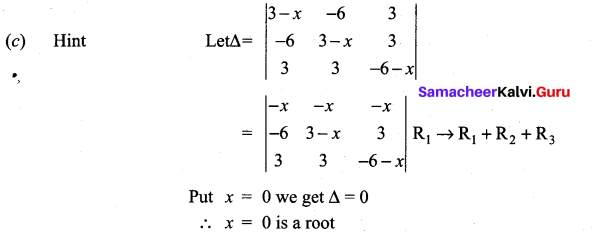Question 17.
The value of the determinant of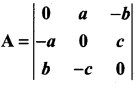is ……………
(a) -2abc
(b) abc
(c) 0
(d) a2 + b2 + c2
Solution: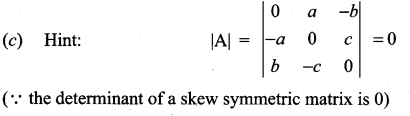Question 18.
If x1, x2, x3 as well as y1, y2, y3 are in geometric progression with the same common ratio, then the points (x1, y1), (x2, y2), (x3, y3) are
(a) vertices of an equilateral triangle
(b) vertices of a right angled triangle
(c) vertices of a right angled isosceles triangle
(d) collinear
Solution:
(d)

Question 19.
If $$\lfloor.\rfloor$$ denotes the greatest integer less than or equal to the real number under consideration and -1 ≤ x < 0, 0 ≤ y < 1, 1 ≤ z ≤ 2, then the value of the determinant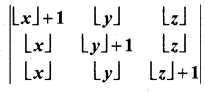is …………..
(a) $$\lfloor z\rfloor$$
(b) $$\lfloor y\rfloor$$
(c) $$\lfloor x\rfloor$$
(d) $$\lfloor x\rfloor+ 1$$
Solution:
(a) Hint: From the given values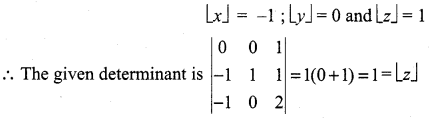>Question 20.
If a ≠ b, b, c satisfy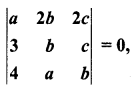then abc = ……………..
(a) a + b + c
(b) 0
(c) b3
(d) ab + bc
Solution:
(c) Hint: Expanding along R1,
a(b2 – ac) – 2b (3b – 4c) + 2c (3a – 4b) = 0
(b2 – ac) (a – b) = 0
b2 = ac (or) a = b
⇒ abc = b(b2) = b3

Question 21.
If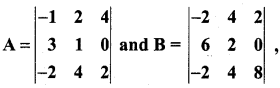then B is given by ………………..
(a) B = 4A
(b) B = -4A
(c) B = -A
(d) B = 6A
Solution: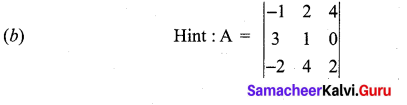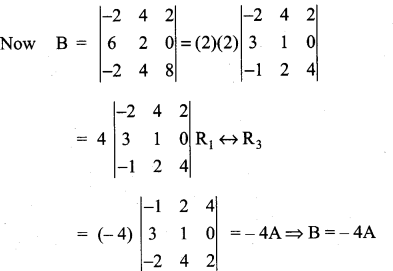Question 22.
IfA is skew-symmetric of order n and C ¡s a column matrix of order n × 1, then CT AC is ……………..
(a) an identity matrix of order n
(b) an identity matrix of order 1
(e) a zero matrix of order I
(d) an Identity matrix of order 2
Solution:
(c) Hint : Given A is of order n × n
C is of order n × 1
so, CT is of order 1 × n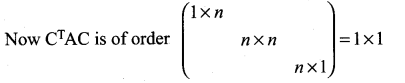Let it be equal to (x) say
Taking transpose on either sides
(CT, AC)T (x)T .
(i.e.) CT(AT)(C) = x
CT(-A)(C) = x
⇒ CTAC = -x
⇒ x = -x ⇒ 2x = 0 ⇒ x = 0

Question 23.
The matrix A satisfying the equation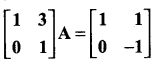is ……………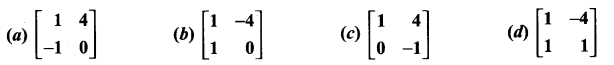Solution: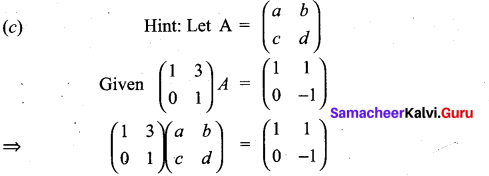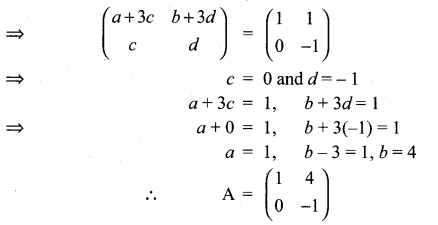Question 24.
If A + I =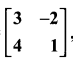, then (A + I) (A – I) is equal to …………….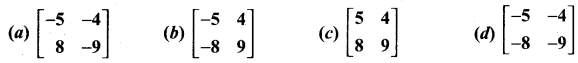Solution: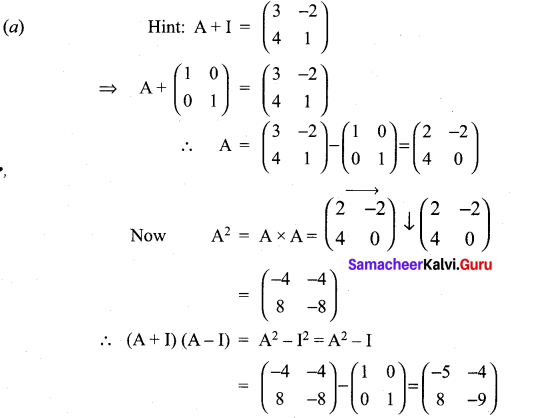Question 25.
Let A and B be two symmetric matrices of same order. Then which one of the following statement is not true?
(a) A + B ¡s a symmetric matrix
(b) AB ¡s a symmetric matrix
(c) AB = (BA)T
(d) ATB = ABT
Solution:
(b)